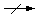Practice Problem 5

Use cell potential data to explain why copper metal does not dissolve in a typical strong acid, such as hydrochloric acid,

 Cu(s) + 2 H+(aq)but will dissolve in 1 M nitric acid.

 3 Cu(s) + 2 HNO3(aq) + 6 H+(aq)3 Cu2+(aq) + 2 NO(g) + 4 H2O(l)

Answer

Copper does not dissolve in a typical strong acid because the overall cell potential for the oxidation of copper metal to Cu2+ ions coupled with the reduction of H+ ions to H2 is negative.

 CuCu2+ + 2 e- Eoox = -(0.34 V) + 2 H+ + 2 e-H2 Eored = 0.000... V ¯¯¯¯¯¯¯¯¯¯¯¯¯¯¯¯¯¯¯¯¯ ¯¯¯¯¯¯¯¯¯¯¯¯¯¯¯¯¯¯¯¯¯ Cu + 2 H+Cu2+ + H2 Eo = Eoox + Eored = -0.34 V

Copper dissolves in nitric acid because the reaction at the cathode now involves the reduction of nitric acid to NO gas, and the potential for this half-reaction is strong enough to overcome the half-cell potential for oxidation of copper metal to Cu2+ ions.

 3 (CuCu2+ + 2 e-) Eoox = -(0.34 V) + 2(HNO3 + 3 H+ + 3 e-NO + 2 H2O) Eored = 0.96 V ¯¯¯¯¯¯¯¯¯¯¯¯¯¯¯¯¯¯¯¯¯¯¯¯¯¯¯¯¯¯¯¯¯¯¯¯¯¯¯¯¯¯ ¯¯¯¯¯¯¯¯¯¯¯¯¯¯¯¯¯¯¯¯¯ 3 Cu + 2 HNO3 + 6 H+3 Cu2+ + 2 NO + 4 H2O Eo = Eoox + Eored = 0.62 V